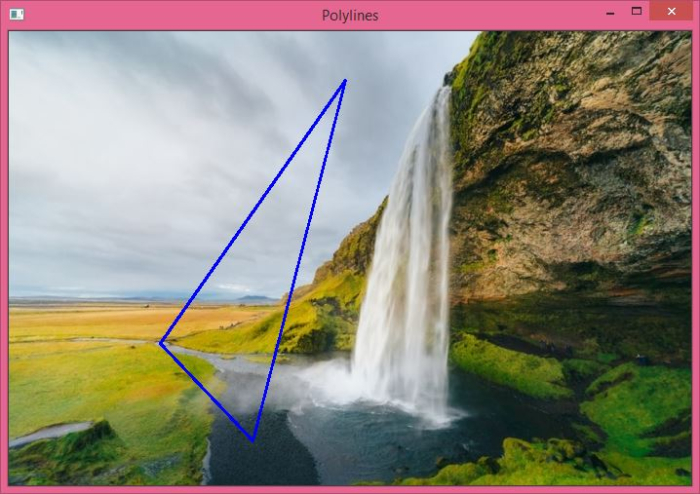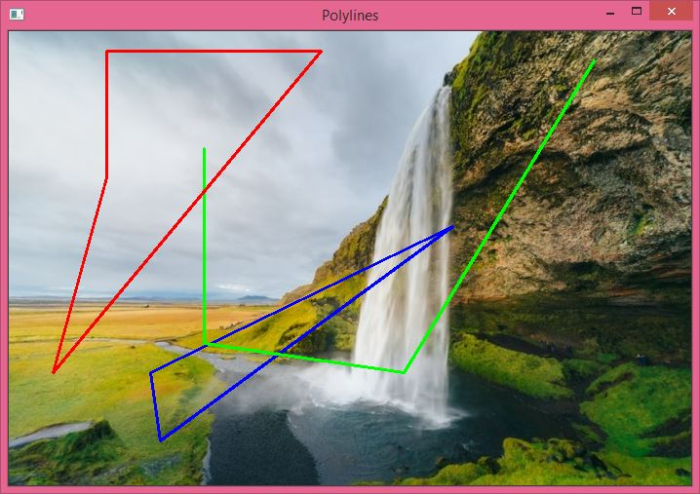# How to draw polylines on an image in OpenCV using Python?

To draw polylines on an image, we use the method cv2.polylines(). We can draw open or closed polylines on the image. The first and last points are not connected while drawing an open polyline.

## Syntax

The syntax of cv2.polylines() is as follows −

cv2.polylines(src, [pts], isClosed, color, thickness)


## Parameters

• src − It's the input image on which the polylines to be drawn.

• pts − List of the array of pints.

• isClosed − Set isClosed=True to draw a closed polyline, for an open polyline set isClosed=False.

• color − It is the color of the line.

• thickness − Its thickness of the line. Default thickness is set to thickness=1.

## Steps

You can use the following steps to to draw polylines on an image −

Import the required libraries. In all the following Python examples, the required Python libraries are OpenCV and NumPy. Make sure you have already installed them.

import cv2
import numpy as np


img = cv2.imread('nature_waterfall.jpg')


Define a 2D array of the points and reshape it.

points = np.array([[155,320],[250,420],[345,50]])
pts = points.reshape(-1,1,2) # now shape [3,1,2]


Draw the polyline on the image passing desired values of the argument− isClosed, color, thickness. To draw a closed polyline, pass isClosed=True and for an open polyline, set isClosed=False.

cv2.polylines(img, [pts1], isClosed=True, color=(255,0,0), thickness = 2)


Display the image with drawn polylines.

cv2.imshow("Polylines", img_poly)
cv2.waitKey(0)
cv2.destroyAllWindows()


We will use this image as the Input File in the following examples.## Example 1

In the Python program below, we have drawn a blue-color closed polyline on the input image.

# import required libraries
import cv2
import numpy as np

# define an array of three points on image to draw the polylines
# shape of point array [3,2]
points = np.array([[155,320],[250,420],[345,50]])

# reshape the point array to make it 3D
pts = points.reshape(-1,1,2) # now shape [3,1,2]

# draw polylines on the image, passing desired values of the arguments
img_poly = cv2.polylines(img, [pts], isClosed=True, color=(255,0,0), thickness = 2)

# display the image with drawn polylines
cv2.imshow("Polylines", img_poly)
cv2.waitKey(0)
cv2.destroyAllWindows()


## Output

When you execute the above program, it will produce the following output window.Notice that the polyline drawn on the image is closed. We can draw a polyline which is not closed. In this case, the first and last points are not joined. Have a look at the second example for both types of lines drawn on the image.

## Example 2

In the Python program below, we draw three polylines on the input image. First and third polylines are closed, whereas the second polyline is not closed.

import cv2
import numpy as np

# define the array of points
points1 = np.array([[455,200],[155,420],[145,350]])
points2 = np.array([[200,120],[200,320],[405,350], [600,30]])
points3 = np.array([[100,20],[320,20],[45,350], [100,150]])

# reshape the points
pts1 = points1.reshape(-1,1,2)
pts2 = points2.reshape(-1,1,2)
pts3 = points3.reshape(-1,1,2)

# draw the polylines
cv2.polylines(img, [pts1], isClosed=True, color=(255,0,0), thickness = 2)
cv2.polylines(img, [pts2], isClosed=False, color=(0,255,0), thickness = 2)
cv2.polylines(img, [pts3], isClosed=True, color=(0,0,255), thickness = 2)

# display the image with drawn polylines
cv2.imshow("Polylines", img)
cv2.waitKey(0)
cv2.destroyAllWindows()


## Output

When you execute the above program, it will produce the following output window.Note that the green polyline is open, whereas the red and blue polylines are closed.